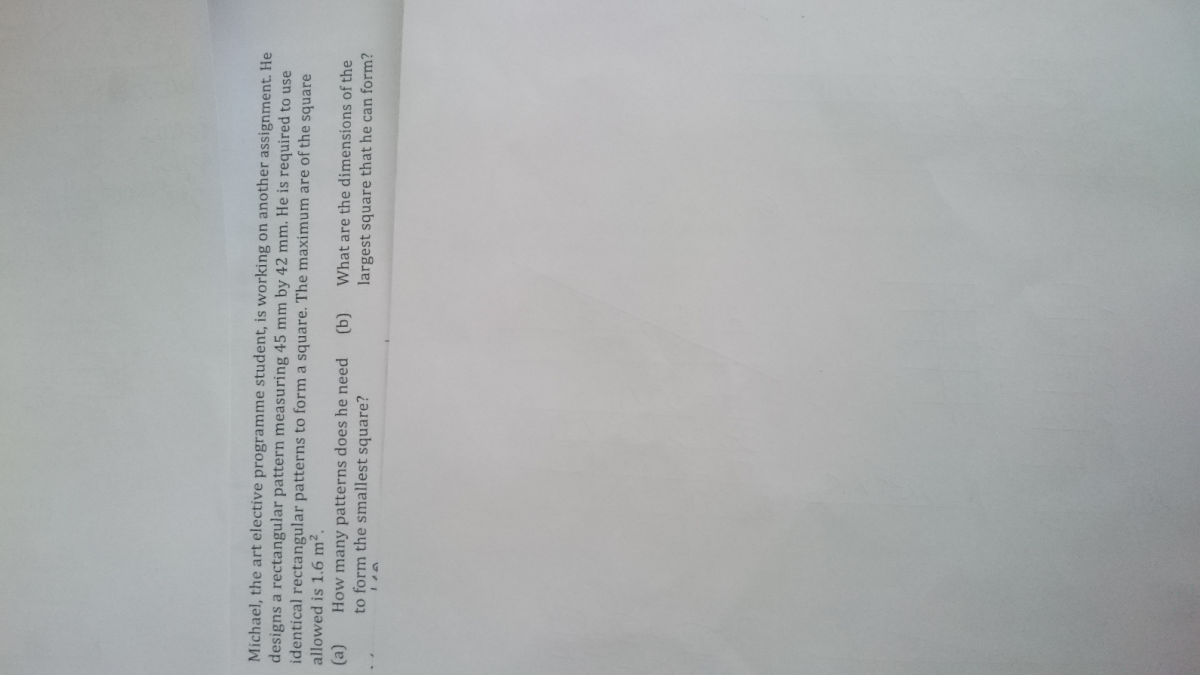# QuestionSorry, don’t know how to flip.

This is a factor question.

a)

45 = 3 × 3 × 5

42 = 3 × 3 × 7

LCM = 3 × 3 × 5 × 7 = 315

Length = 315 ÷ 45 = 7

Breadth = 315 ÷ 42 = 5

7 × 5 = 35 patterns to make a square

b)

Length = sqrt (1.6 × 1000 × 1000) = 1264 mm

1264 ÷ 315 = 4

4 × 315 = 1260 mm = 1.26 m

1.26 m by 1.26 m

1 Reply 0 Likes

Thank you for your solutions, Khong Pek Mao.

I have not received the solutions from ds teacher, i will update once he reviews in class.  Thank you for your input.  Greatly appreciate it.

0 Replies 0 Likes